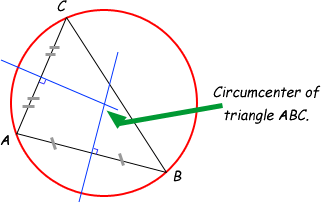SEARCH HOMEMath Central Quandaries & QueriesQuestion from Kathryn, a student: I cannot figure out how to do the question below, as my book does not explain it clearly. It would be a huge help if you could show me how to do it. Thanks in advance, Kathryn. Q1. Find the coordinates of the circumcentre of the triangle whose vertices are: (5, -3), (3, -1), (-1, 5)Hi Kathryn.

According to our glossary, the circumcenter of a triangle is the point which is the center of a circle that includes the vertices of the triangle on its circumference.

Thus, each side of the triangle is a chord of the circle. Since the perpendicular bisector of a chord is a line that goes through the center of the circle, you need to find the equations of the perpendicular bisectors of two of the sides and then find the intersection of those two lines.Choose two sides and use the midpoint formula to find their midpoints. Then determine the slopes of these same sides and from those, the slopes of lines perpendicular to those sides. This gives you enough information to write the two equations of the perpendicular bisectors. Then you can find the intersection which is the circumcentre (circumcenter).

Cheers,
Stephen La Rocque.Math Central is supported by the University of Regina and The Pacific Institute for the Mathematical Sciences.